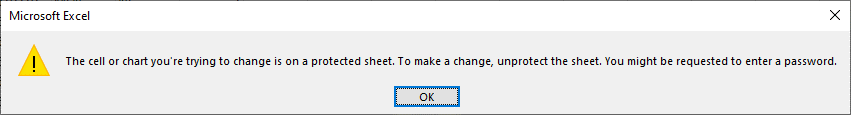Search

# vba code to unprotect Excel worksheets

On the Review tab of Excel, you will find the option to password protect a worksheet. [Note that if you choose the option to protect the workbook, this will only prevent worksheets and other structural elements of the Excel file from being changed or removed. It will not prevent the data on a worksheet from being copied and edited.} It's not uncommon to come across Excel files in document productions that have password protected worksheets. When you try to select data on the worksheet, you'll get this message:Excel does not use strong encryption, and most passwords can be cracked using the VBA code posted here, and copied below. The macro will actually change the password to a sequence of As and Bs, and not reveal the actual password. The new password will be displayed in a dialog box. Click OK, and then the worksheet will be fully editable.I tested this macro tonight on a workbook protected with Excel 2019 using a four-digit number, a dictionary word, a short phrase, and an eight character alphanumeric code and it cracked each one in seconds.

Dim i As Integer, j As Integer, k As Integer

Dim l As Integer, m As Integer, n As Integer

Dim i1 As Integer, i2 As Integer, i3 As Integer

Dim i4 As Integer, i5 As Integer, i6 As Integer

On Error Resume Next

For i = 65 To 66: For j = 65 To 66: For k = 65 To 66

For l = 65 To 66: For m = 65 To 66: For i1 = 65 To 66

For i2 = 65 To 66: For i3 = 65 To 66: For i4 = 65 To 66

For i5 = 65 To 66: For i6 = 65 To 66: For n = 32 To 126

ActiveSheet.Unprotect Chr(i) & Chr(j) & Chr(k) & _

Chr(l) & Chr(m) & Chr(i1) & Chr(i2) & Chr(i3) & _

Chr(i4) & Chr(i5) & Chr(i6) & Chr(n)

If ActiveSheet.ProtectContents = False Then

MsgBox "Password is " & Chr(i) & Chr(j) & _

Chr(k) & Chr(l) & Chr(m) & Chr(i1) & Chr(i2) & _

Chr(i3) & Chr(i4) & Chr(i5) & Chr(i6) & Chr(n)

Exit Sub

End If

Next: Next: Next: Next: Next: Next

Next: Next: Next: Next: Next: Next

End Sub